## Capillary

Capillary action is the physical phenomenon arising due to surface tension on the interface of immiscible media. Commonly, capillary phenomena occur in liquid media and are brought about by the curvature of their surface that is adjacent to another liquid, gas, or its own vapor.
Surface curvature in a fluid gives rise to an additional so-called capillary pressure ρσ whose value is related to an average surface curvature H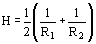(R1 and R2 are the radii of curvature of principal normal sections) via the Laplace equation.
(1)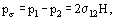where σ12 is the surface or interfacial tension (see Surface and Interfacial Tension) on the boundary between phases 1 and 2, p1 and p2 are the pressures in the phases. The pressure is higher in the phase to which the concavity of the interface is presented. For a plane interface, H=0 and pσ=0.
Capillary phenomena include various cases of equilibrium and flow of fluid surface under the action of surface tension forces and of external forces, primarily gravity. In the simplest case when the external forces are absent, e.g., under weightlessness conditions, a limited fluid volume takes the shape of a sphere due to surface tension forces. This state corresponds to a stable equilibrium of the fluid since a sphere has the minimum surface and, consequently, the minimum surface energy.
As shown in Figure 1, when the liquid (1) comes in contact with the gas vapor (2) and the solid (3), the shape of the free liquid surface depends on wetting. Interaction of surface tension forces at the liquid-gas σ12, liquid-solid σ13, and gas-solid σ23 interfaces is responsible for a curved area of the liquid surface (meniscus) near the solid. An angle θ made by the tangent to the liquid surface and the solid surface, known as a wetting angle, is determined by the balance of forces within the surface tension. According to the Young equation,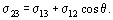If σ23 > σ13, i.e., if the surface tension between gas and a solid is greater than between a solid and a liquid, then θ < π/2 and the solid surface is said to be wetted by the liquid. If σ23 < σ13, then θ > π/2, and this indicates that the solid is not wetted by the liquid. The cases θ = 0 and θ = π correspond to absolute wetting and nonwetting of the surface by the liquid.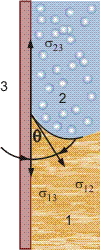Figure 1. Solid surface in contact with gas and liquid gases.
The wetting angle depends on the liquid and the surface it is in contact with. For instance, liquid alkaline metals and cryogenic liquids wet metal surfaces nearly absolutely and the angle θ approaches zero. Teflon and paraffin are virtually nonwettable by water and some other liquids. It should be borne in mind that the wetting angle strongly depends on the surface state, i.e., surface contamination—the presence of surface active agents—and surface roughness, which enhances wettability.
As a result of displacement of the interface of the three phases, the wetting angle displays hysteresis; that is, on the surface wetted earlier, the wetting angle appears to be smaller than in the case of displacement of the interphase boundary on an initially dry surface.
A most commonly encountered and visual example of capillary phenomena are the suction of liquid in narrow tubes with wettable walls (Figure 2a) and the expulsion of the liquid out of them if the walls are nonwettable (Figure 2b). At each point of the curved free liquid surface, capillary pressure is built up that leads to a liquid rise in the tube. The height of rise h is determined by the relation between external (in this case, gravitational) and surface tension forces. This relation is expressed by the Bond Number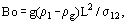where p1 and pg are the densities of the liquid and gaseous (vapor) phases, respectively, and L, the characteristic linear dimension of interface. The condition Bo=1 governs the linear dimension of a at which the indicted forces are equal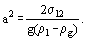a2, known as the capillary constant, is a constant of the liquid-vapor or liquid 1-liquid 2 interface which is independent of the tube diameter and material its wall is made of. Under terrestrial conditions, at pressures substantially lower than critical a=1−3 mm for most liquids.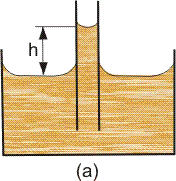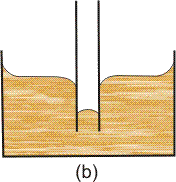Figure 2. Level rise and depression due to capillary action.
Tubes with diameter dc<a are said to be capillary. If the capillary radius rc<0.05 a, then the meniscus has the shape close to a sphere with the curvature radius
(2)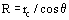and the height h of the liquid is determined by Jurin's formula
(3)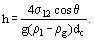As rc increases, the meniscus shape steadily departs from the spherical and the capillary rise also steadily deviates from the value calculated via Eq. (3). Rayleigh suggested a formula in the form of a power series which allows for this effect, and is valid for rc<0.46 a: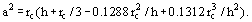The terms on the right-hand side, beginning with the second, are the correction for deviation of the meniscus shape from the sphere.
In very narrow (dc << a) capillary tubes, the vapors of an absolutely wetting liquid absorbing on the walls form a multimolecular liquid film. The presence of the film decreases the curvature radius of the meniscus as compared to that calculated through Eq. (2) at θ=0. In this case, the capillary pressure on the meniscus exceeds the value calculated by the Laplace equation (1) at R1=R2=rc.
In a plane capillary tube, i.e., the gap between two plates at distance dp apart, the meniscus surface is of cylindrical shape with the radius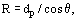therefore, the height of the capillary rise is half that in a cylindrical capillary at dc=dp.
If the capillary walls are not wetted, the liquid meniscus is convex and the level of liquid in it deviates below the level of a free liquid by a value also determined by Eq. ( 3).
In vessels with the characteristic dimension L>>a, the liquid surface is plane except the small area near the vessel walls, where the liquid rises or lowers by about the quantity a.
The difference in capillary pressures due to different curvature of liquid menisci may give rise to a liquid flow in the capillary (Figure 3), where the flow of wetting liquid is directed toward the meniscus with the smaller curvature radius. This is used, for instance, when entraining the working liquid from the condensation to the evaporation zone in heat pipes. (See Heat Pipes.) The capillary pressure can be calculated theoretically only for capillaries of the simplest form: a plane slot which has been considered above; a circular cylindrical capillary; and capillaries of triangular, square, and some other shapes of cross-section. For capillaries with cross-sections of intricate irregular shape (such as the case, in particular, with capillary paths of heat pipe wicks), an accurate value of capillary pressure can be found only experimentally.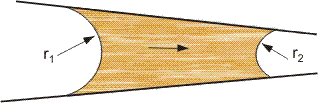Figure 3. Difference in radius of curvature inducing flow in a capillary.
Capillary absorption plays an essential role in liquid movement in the soil, ground and porous coatings of heat-releasing surface with boiling liquids on them. Capillary impregnation of materials is widely applied in chemical technology.
Many properties of disperse systems, such as permeability and strength, depend to a large extent on capillary phenomena because in the fine pores of these bodies, a high capillary pressure is realized.
At a given temperature T, the intermolecular cohesive forces in a concave surface layer are stronger than on the plane surface. Therefore, the number of molecules leaving the surface and, as a result, the saturation pressure over the concave surface, is lower than those over the plane surface. For a convex surface, this pressure is higher. The relation between pressures p and p0 over curved and plane surfaces, respectively, is described by the Kelvin equation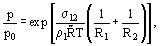where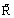is the individual gas constant and pl, the liquid density.
In narrow pores, the vapors of wetting liquid are condensed at the temperature of vapor saturation over the plane surface (capillary condensation). This underlies vapor collecting by fine-pored sorbents.
Curvature of a free liquid surface exposed to external forces gives rise to so-called capillary waves, that is, the rippled surface of liquid.
The joint action of surface tension, viscosity, inertia, and gravitational forces and their correlation account for the diverse flow regimes, i.e., phase distribution throughout the volume in Two-Phase Flows in channels.
The measurement of some physical values characterizing capillary phenomena forms the basis for experimental methods determining surface tension coefficients of liquids. For instance, in measuring the height h of liquid rise in the capillary Eq. (3) is used to find the surface tension coefficient σ12.
Leonardo da Vinci (16th century), B. Pascal (17th century) and Jurin (18th century) pioneered investigations into capillary phenomena in experiments with capillary tubes. The theory of capillary phenomena was further developed by P. Laplace (1806), T. Young (1879) and I. S. Gromeka (1876).